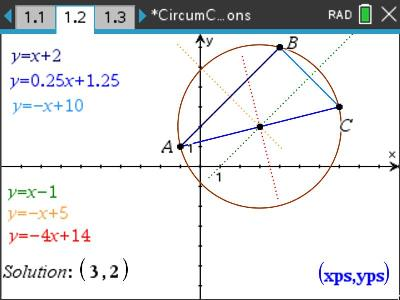•• ##### Device
•TI-Nspire™ CX
•TI-Nspire™ CX CAS
• ##### Software

TI-Nspire™
TI-Nspire™ CAS

4.5

# QLD (Nspire): Circumcircles

by Texas Instruments#### Objectives

Students are required to to determine the following:

• Equation to a straight line given two points.
• Equation to a straight line given the gradient (perpendicular) and a point.
• Point of intersection between two or more lines.
• Equation to a circle given centre and radius

#### Vocabulary

• Circumcentre
• Circumcircle
• Perpendicular bisector
• Simultaneous equations